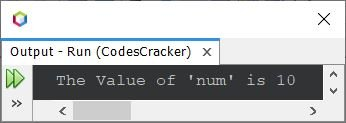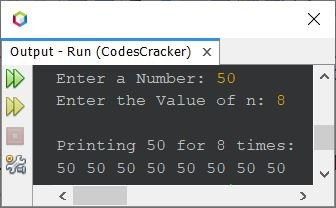# Java Program to Print Integers

## Print a Number in Java

The question is, write a Java program to print a number on the output screen. The program given below is its answer:

```import java.util.Scanner;

public class CodesCracker
{
public static void main(String[] args)
{
int num=10;
System.out.println("\nThe Value of 'num' is " +num);
}
}```

The snapshot given below shows the output produced by this Java program, on printing of a number:## Print a Number in Java using for Loop

This program is created to print a number entered by user at run-time of the program, for n number of times using for loop. The value of n also get received by user at run-time.

```import java.util.Scanner;

public class CodesCracker
{
public static void main(String[] args)
{
Scanner scan = new Scanner(System.in);

System.out.print("Enter a Number: ");
int num = scan.nextInt();
System.out.print("Enter the Value of n: ");
int n = scan.nextInt();

System.out.println("\nPrinting " +num+ " for " +n+ " times:");
for(int i=0; i<n; i++)
System.out.print(num+ " ");
}
}```

Here is its sample run with user input 50 as number to print, and 8 as the value of n to print 50 for 8 times:Java Online Test

« Previous Program Next Program »